# Basics of Disjoint Data Structures

The efficiency of an algorithm sometimes depends on the data structure that is used. An efficient data structure, like the disjoint-set-union, can reduce the execution time of an algorithm.

Assume that you have a set of N elements that are into further subsets and you have to track the connectivity of each element in a specific subset or connectivity of subsets with each other. You can use the union-find algorithm (disjoint set union) to achieve this.

Let%u2019s say there are 5 people A, B, C, D, and E.
A is B's friend, B is C's friend, and D is E's friend, therefore, the following is true:

1. A, B, and C are connected to each other
2. D and E are connected to each other

You can use the union-find data structure to check each friend is connected to the other directly or indirectly. You can also determine the two different disconnected subsets. Here, 2 different subsets are {A, B, C} and {D, E}.

You have to perform two operations:

1. Union(A, B): Connect two elements A and B
2. Find(A, B): Find whether the two elements A and B are connected

Example You have a set of elements S = {0, 1, 2, 3, 4, 5, 6, 7, 8, 9}. Here there are 10 elements (N = 10). Use an array arr to manage the connectivity of the elements. Arr[ ] that is indexed by elements of sets, which are of size N (as N elements in set), can be used to manage the operations of union and find.

Assumption

A and B objects are connected only if arr[ A ] = arr[ B ].

Implementation

To implement the operations of union and find, do the following:

1. Find(A, B): Check whether arr[ A ] = arr[ B ]
2. Union(A, B): Connect A to B and merge the components that comprise A and B by replacing elements that have a value of arr[ A ] with the value of arr[ B ].

Initially there are 10 subsets and each subset has one element in it.When each subset contains only one element, the array arr is as follows: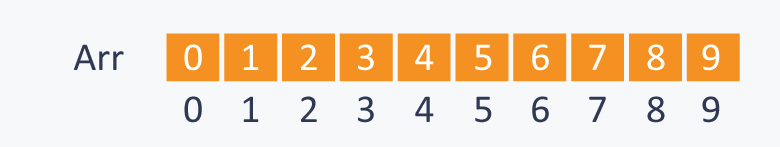Let%u2019s perform some operations.

1. Union(2, 1)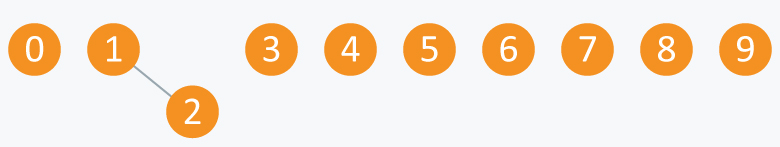The arr is as follows: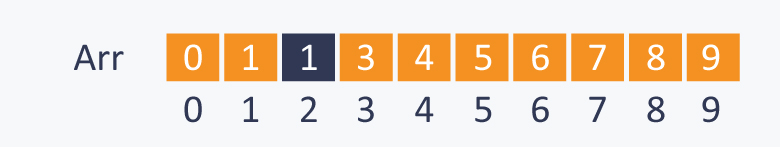1. Union(4, 3)

2. Union(8, 4)

3. Union(9, 3)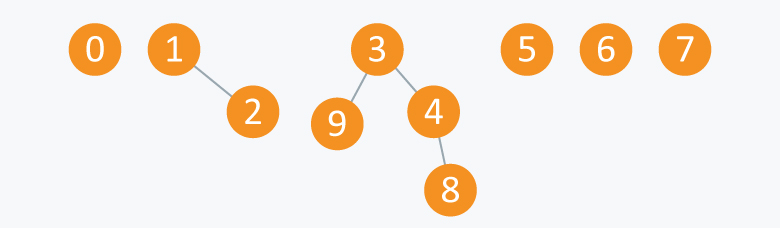The arr is as follows: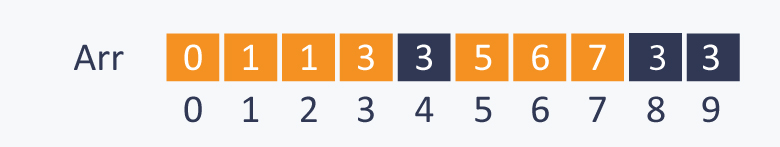1. Union(6, 5)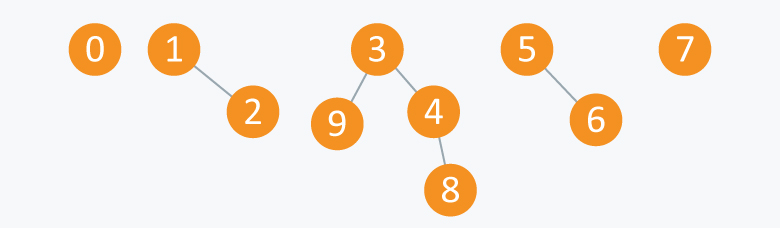The arr is as follows: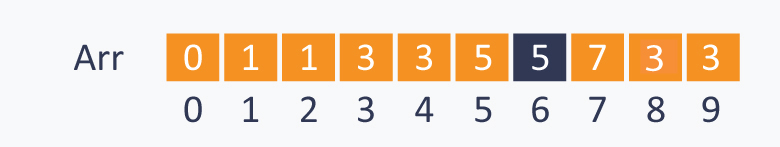After performing some operations of Union (A ,B), there are now 5 subsets as follows:

1. First subset comprises the elements {3, 4, 8, 9}
2. Second subset comprises the elements {1, 2}
3. Third subset comprises the elements {5, 6}
4. Fourth subset comprises the elements {0}
5. Fifth subset comprises the elements {7}

The elements of a subset, which are connected to each other directly or indirectly, can be considered as the nodes of a graph. Therefore, all these subsets are called connected components.

The union-find data structure is useful in graphs for performing various operations like connecting nodes, finding connected components etc.

Let%u2019s perform some find(A, B) operations.

1. Find(0, 7): 0 and 7 are disconnected, and therefore, you will get a false result
2. Find(8, 9): Although 8 and 9 are not connected directly, there is a path that connects both the elements, and therefore, you will get a true result

When these operations are viewed in terms of components, then:

1. Union(A, B): Replace components that comprise the two objects A and B with their union
2. Find(A, B): Check whether the two objects A and B are in the same component

If you perform the operation union(5, 2) on the components mentioned above, then it will be as follows: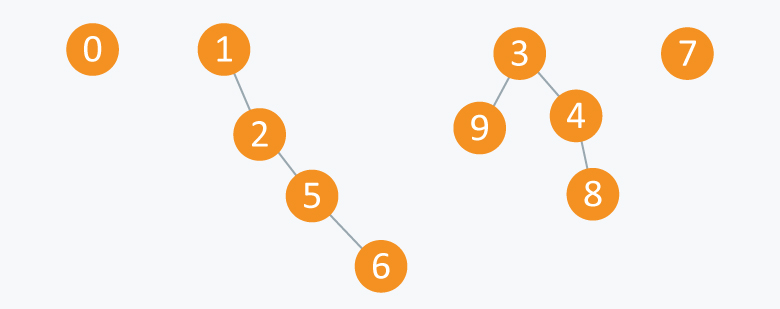The arr is as follows: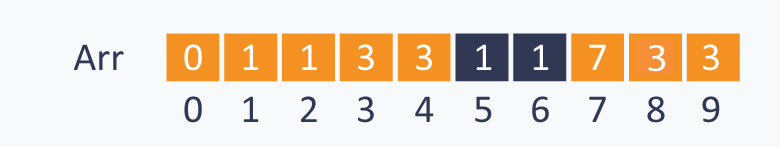Implementation

Approach A

Initially there are N subsets containing one element in each subset. Therefore, to initialize the array use the initialize () function.

``````    void initialize( int Arr[ ], int N)
{
for(int i = 0;i<N;i++)
Arr[ i ] = i ;
}
//returns true if A and B are connected, else returns false
bool find( int Arr[ ], int A, int B)
{
if(Arr[ A ] == Arr[ B ])
return true;
else
return false;
}
//change all entries from arr[ A ] to arr[ B ].
void union(int Arr[ ], int N, int A, int B)
{
int TEMP = Arr[ A ];
for(int i = 0; i < N;i++)
{
if(Arr[ i ] == TEMP)
Arr[ i ] = Arr[ B ];
}
}``````

Time complexity (of this approach)

As the loop in the union function iterates through all the N elements for connecting two elements, performing this operation on N objects will take O(N2) time, which is quite inefficient.

Implementation Code:

#include<iostream>
using namespace std;
void initialize( int Arr[ ], int N)
{
for(int i = 0;i<N;i++)
Arr[ i ] = i ;
}
//returns true if A and B are connected, else returns false
bool find( int Arr[ ], int A, int B)
{
if(Arr[ A ] == Arr[ B ])
return true;
else
return false;
}
//change all entries from arr[ A ] to arr[ B ].
void unionr(int Arr[ ], int N, int A, int B)
{
int TEMP = Arr[ A ];
for(int i = 0; i < N;i++)
{
if(Arr[ i ] == TEMP)
Arr[ i ] = Arr[ B ];
}
}
int main(){
int a,n=10;
initialize(a,n);
cout<<"Printing array entries:\n";
for(int i=0;i<n;i++){
cout<<a[i]<<" ";
}
cout<<endl;
cout<<"Checking whether 2 and 3 belongs to same array:\n";
if(!find(a,2,3)){
cout<<"No";
}
else{
cout<<"Yes";
}
cout<<endl;
cout<<"Making 2 and 3 to be in same group\n";
unionr(a,n,2,3);
cout<<"Checking whether 2 and 3 belongs to same array:\n";
if(!find(a,2,3)){
cout<<"No";
}
else{
cout<<"Yes";
}
cout<<endl;

}
--------------------------------------------------
Output:
```Printing array entries:
0 1 2 3 4 5 6 7 8 9
Checking whether 2 and 3 belongs to same array:
No
Making 2 and 3 to be in same group
Checking whether 2 and 3 belongs to same array:
Yes```#### More Articles of M Mounika:

Name Views Likes
C++ Segmented Sieve (Print Primes In a Range) 162 0
C++ Sieve Of Erastosthenes 135 0
C++ Gold Mine Problem 294 0
C++ Merge K Sorted Arrays 116 0
C++ K Centers Problem 239 0
C++ Find Nth Catalan Number 311 0
C++ Inplace Rotate square matrix by 90 degrees 285 0
C++ Find Non Repeating Elements in Array 86 0
C++ Merge Two Binary Trees 120 0
C++ Sum of Numbers From Root To Leaf Paths 88 0
C++ Meta Strings 91 0
C++ Flood Fill Algorithm 402 0
C++ smallest substring with maximum distinct characters 199 0
C++ Smallest window with all characters in string 93 0
C++ Minimum Removal of Characters from string to make its permutation as palindrome 86 0
C++ Minimum characters added at front of string in palindrome conversion 69 0
C++ Number of Bracket Reversals needed to make expression Balanced 72 0
C++ String to Palindrome with Append Function 83 0
C++ WildCard pattern matching 75 0
C++ Anagram substring Search 71 0
C++ Manachars Algorithm 74 0
C++ Search String in Grid 83 0
C++ String Matching(Z Algorithm) 67 0
C++ String Matching(Naive Algorithm) 113 0
C++ String Matching(KMP Algorithm) 140 0
C++ Remove Duplicates From String 110 0
C++ Basics of String Manipulation 84 1
C++ Disjoint Data Structure Cycle Detection 86 0
C++ Problem On Disjoint Data Structures 94 0
C++ Disjoint Data Structures Part3 78 0
Disjoint Data Structures Part2 90 0
Disjoint Data Structures 93 1
C++ Segment Trees 321 2
C++ Trie Cost of Data 290 1
C++ Trie Datastructure 278 1
C++ Greedy Approach Minimum number of coins 525 0
C++ Greedy Approach Maximum height Pyramid 328 1
C++ Greedy Approach String lexicographically largest subsequence 246 0
C++ Greedy Approach Lexicographically largest subsequence 364 0
C++ Greedy Approach Prims MST 398 1
C++ Greedy Approach Krushkals MST 457 1
C++ Greedy Approach N-array maximum sum 333 1
C++ Greedy Approach Policemen Catch Thieves 563 1
C++ Greedy Approach Maximum product Subset 545 1
C++ Greedy Approach Minimum Product Subset 348 1
C++ Greedy Approach Fractional Knapsack 737 1
C++ Greedy Approach-Activity Selection Problem 744 1
C++ Greedy Approach-Egyptian Fractions 639 0
C++ Greedy Approach-Huffman Codes 1030 1
C++ Introduction to Greedy Approach 955 2